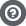Hello Guest

# Yet another projection matrix problem

• 4 Replies
• 34 Views####CDnoMlqko

•• 4##### Yet another projection matrix problem
« on: September 29, 2020, 13:59:15 »
Hello!

I am really sorry if this question is stupid.

So, I am developing a 3D game engine and I need to calculate the projection matrix for the camera. Currently, I am doing it this way:
Code: [Select]
`viewMatrix.identity()   .rotateX(transform.getRotation().x)   .rotateY(transform.getRotation().y)   .rotateZ(transform.getRotation().z)   .translate(new Vector3f(transform.getPosition()).mul(-1));`where "transform" is a data container for the position and rotation of the camera. And it works. But, as I am calling this every frame, I think there should be a faster solution.
I know that the view matrix should be the inverse of the camera world matrix. So I tried this:
Code: [Select]
`transform.getWorldMatrix().invert(viewMatrix);`, where getWorldMatrix() looks like this:
Code: [Select]
`worldMatrix.translation(position).rotateX(rotation.x).rotateY(rotation.y).rotateZ(rotation.z).scale(scale);`which should write the inverse of the world matrix in the view matrix, but the result of that is some quite confusing. It looks like all objects are rotating around the world (0,0,0), but I am not sure if exactly this is the case.####KaiHH

•• 283##### Re: Yet another projection matrix problem
« Reply #1 on: September 29, 2020, 16:34:24 »
Well, the inverse of:

Code: [Select]
`  Rx * Ry * Rz * T`
is:

Code: [Select]
`  T^-1 * Rz^-1 * Ry^-1 * Rx^-1`
where T^-1 is a translation by the negated vector
and Ra^-1 is a rotation by the negated angle around axis 'a'

Also note the inverted order of the transformations.

So, the inverse of worldTransform _would_ be the same as your current viewMatrix, if worldTransform were:

Code: [Select]
`worldMatrix.translation(position).rotateZ(-rotation.z).rotateY(-rotation.y).rotateX(-rotation.x);`####CDnoMlqko

•• 4##### Re: Yet another projection matrix problem
« Reply #2 on: September 29, 2020, 17:56:00 »
So, you say that my worldMatrix calculation is wrong? In short: how can I make it work?####CDnoMlqko

•• 4##### Re: Yet another projection matrix problem
« Reply #3 on: September 29, 2020, 19:07:37 »
OK, I fixed it!

this is the worldmatrix code:
Code: [Select]
`worldMatrix.translation(this.position) .rotateZ(this.rotation.z) .rotateY(this.rotation.y) .rotateX(this.rotation.x) .scale(this.scale);`
and this is the viewMatrix code:
Code: [Select]
`transform.getWorldMatrix().invert(viewMatrix);`Thank you, @khaihh####KaiHH

•• 283##### Re: Yet another projection matrix problem
« Reply #4 on: September 29, 2020, 19:21:11 »
That's great!
Btw.: If you want to cut down a few nanoseconds for copying unused fields internally in the matrix, you can use Matrix4x3f instead of Matrix4f for affine transformations.
All affine transformations have the same name on a Matrix4x3f as they have on a Matrix4f and they multiply with Matrix4x3f and Matrix4f.
Just beware when/if you put that matrix into a memory location expecting a 4x4 matrix: In that case it's not matrix4x3.get(bufferOrArray) but matrix4x3.get4x4(bufferOrArray).